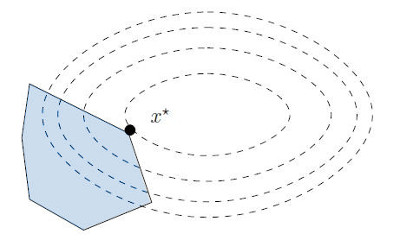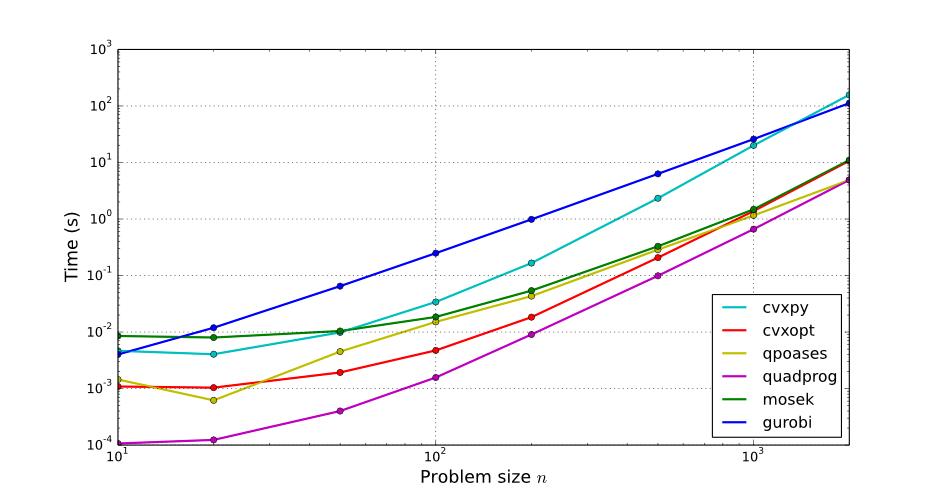# Quadratic programming in Python

Quadratic programs are a class of numerical optimization problems with wide-ranging applications, from curve fitting in statistics, support vector machines in machine learning, to inverse kinematics in robotics. They are the first step beyond linear programming in convex optimization. We will now see how to solve quadratic programs in Python using a number of available solvers: CVXOPT, CVXPY, Gurobi, MOSEK, qpOASES and quadprog.

## Standard form of quadratic programs

A quadratic program (QP) is written in standard form as:

\begin{equation*} \begin{array}{rl} \mathrm{minimize} & (1/2) x^T P x + q^T x \\ \mathrm{subject\ to} & G x \leq h \\ & A x = b \end{array} \end{equation*}

Here $$x$$ is the vector of optimization variables $$x_1, \ldots, x_n$$. The matrix $$P$$ and vector $$q$$ are used to define a general quadratic objective function on these variables, while the matrix-vector pairs $$(G, h)$$ and $$(A, b)$$ respectively define inequality and equality constraints. Vector inequalities apply coordinate by coordinate, so that for instance $$x \geq 0$$ means that every coordinate of the vector $$x$$ is positive.

This mathematical formulation means that a QP finds the minimum of a quadratic function over a linear set:In the 2D illustration above, the level sets of the quadratic function are drawn as dashed ellipses while the linear set of inequality constraints corresponds to the blue polygon. (The description of a polygon, or more generally a polyhedron, by linear inequality constraints is called the halfspace representation.) Since the global optimal of the objective function is outside of the polygon, the solution $$x^*$$ of the QP lies on the boundary of this polygon. The set of linear constraints that are saturated at $$x^*$$ is called the active set, but that's a story for another post...

Back to the standard form, you will notice that there is no constant term in the objective function. Indeed, it would have no effect on the result of the optimization, which is the location of the solution $$x^*$$. For example, the quadratic expression $$\| A x - b \|^2$$ of a least squares optimization is written in standard form with $$P = 2 A^T A$$ and $$q = -2 A^T b$$ (see the example below for a small proof of this).

The standard form also assumes, without loss of generality, that the matrix $$P$$ is symmetric. Any matrix $$M$$ can be decomposed as sum of its symmetric part $$M^+$$ and antisymmetric part $$M^-$$, and the latter yields zero in $$x^T M^- x$$. Note that some solvers (like CVXOPT) assume that you provide a symmetric cost matrix right away: they won't check this, and will return wrong results if you don't.

## Setting up QP solvers

Two readily-available QP solvers in Python are CVXOPT and quadprog. They can be installed by:

$sudo CVXOPT_BUILD_GLPK=1 pip install cvxopt$ sudo pip install quadprog


CVXOPT uses its own matrix type, and it requires the matrix $$P$$ of the objective function to be symmetric. To be on the safe side, you can wrap it as follows:

def cvxopt_solve_qp(P, q, G, h, A=None, b=None):
P = .5 * (P + P.T)  # make sure P is symmetric
args = [cvxopt.matrix(P), cvxopt.matrix(q)]
args.extend([cvxopt.matrix(G), cvxopt.matrix(h)])
if A is not None:
args.extend([cvxopt.matrix(A), cvxopt.matrix(b)])
sol = cvxopt.solvers.qp(*args)
if 'optimal' not in sol['status']:
return None
return numpy.array(sol['x']).reshape((P.shape,))


The quadprog module works directly on NumPy arrays so there is no need for type conversion. Its matrix representation is equivalent to the standard form but combines inequalities and equalities in a single matrix-vector pair:

def quadprog_solve_qp(P, q, G, h, A=None, b=None):
qp_G = .5 * (P + P.T)   # make sure P is symmetric
qp_a = -q
if A is not None:
qp_C = -numpy.vstack([A, G]).T
qp_b = -numpy.hstack([b, h])
meq = A.shape
else:  # no equality constraint
qp_C = -G.T
qp_b = -h
meq = 0
return quadprog.solve_qp(qp_G, qp_a, qp_C, qp_b, meq)


In these two functions we assume that the QP has inequality constraints. For more general functions that handle all combinations of inequality, equality and box-inequality constraints $$lb \leq x \leq ub$$, or if you want to try out other solvers, you will find a unified solve_qp function with a solver keyword argument in the qpsolvers library.

## Example of a linear least squares problem

For a small example, let us see how to solve:

\begin{align*} \begin{array}{rl} \underset{x_1, x_2, x_3}{\mathrm{minimize}} & \left\| \left[\begin{array}{ccc} 1 & 2 & 0 \\ -8 & 3 & 2 \\ 0 & 1 & 1 \end{array}\right] \left[\begin{array}{c} x_1 \\ x_2 \\ x_3\end{array}\right] - \left[\begin{array}{c} 3 \\ 2 \\ 3\end{array}\right] \right\|^2 \\ \mathrm{subject\ to} & \left[\begin{array}{ccc} 1 & 2 & 1 \\ 2 & 0 & 1 \\ -1 & 2 & -1 \end{array}\right] \left[\begin{array}{c} x_1 \\ x_2 \\ x_3\end{array}\right] \leq \left[\begin{array}{c} 3 \\ 2 \\ -2 \end{array} \right] \end{array} \end{align*}

This problem is in linear least squares form. Denoting its cost function by $$\| M x - b\|^2$$, we can convert it to QP form as follows:

\begin{equation*} \begin{array}{rcl} \| M x - b \|_2^2 & = & (M x - b)^T (M x - b) \\ & = & x^T M^T M x - x^T M^T b - b^T M x + b^T b \\ & \propto & (1/2) x^T M^T M x - (1/2) x^T M^T b - (1/2) b^T M x \\ & = & (1/2) x^T (M^T M) x + (-M^T b)^T x \end{array} \end{equation*}

Multiplying by a positive constant $$(1/2)$$ does not change the value of the optimum $$x^*$$. Similarly, the constant offset $$b^T b$$ does not affect $$x^*$$, therefore we can leave it out. Meanwhile, $$y^T = y$$ for any real number $$y$$, therefore $$x^T M^T b = b^T M x$$ and we can combine the two middle terms into a single $$q = -M^T b$$. In Python, we then write:

M = array([[1., 2., 0.], [-8., 3., 2.], [0., 1., 1.]])
P = dot(M.T, M)
q = -dot(M.T, array([3., 2., 3.]))
G = array([[1., 2., 1.], [2., 0., 1.], [-1., 2., -1.]])
h = array([3., 2., -2.]).reshape((3,))


We can finally compute the solution to the least squares problem using either of our QP solvers:

In : cvxopt_solve_qp(P, q, G, h)
Out: array([ 0.12997347, -0.06498674,  1.74005305])

In : quadprog_solve_qp(P, q, G, h)
Out: array([ 0.12997347, -0.06498674,  1.74005305])


## Comparing solver performances

In the following benchmark, I compared six different solvers. Three of them are numerical, which is the approach we have seen so far:

The three others are symbolic, meaning that if you dig into their API they allow you to construct your problem formally (with variable names) rather than using the matrix-vector representation. This is convenient for big sparse problems, but slower and small problems such as the one we are looking at here. The three symbolic frameworks I tested are:

Note that ECOS and MOSEK are actually SOCP solvers, SOCP being a class of problems more general that QP. Here is a sample of computation times on my machine:

• qpOASES: 10000 loops, best of 3: 31.5 µs per loop
• quadprog: 10000 loops, best of 3: 34.1 µs per loop
• CVXOPT: 1000 loops, best of 3: 559 µs per loop
• Gurobi: 1000 loops, best of 3: 865 µs per loop
• CVXPY: 100 loops, best of 3: 2.81 ms per loop
• MOSEK: 100 loops, best of 3: 7.24 ms per loop

For further investigation, let us generate random problems of arbitrary size as follows:

def solve_random_qp(n, solver):
M, b = random.random((n, n)), random.random(n)
P, q = dot(M.T, M), dot(b, M).reshape((n,))
G = toeplitz([1., 0., 0.] + [0.] * (n - 3), [1., 2., 3.] + [0.] * (n - 3))
h = ones(n)
return solve_qp(P, q, G, h, solver=solver)


The Toeplitz matrix used to generate inequalities is just an upper-tridiagonal matrix with coefficients 1, 2, 3, all other coefficients being zero. This matrix is sparse but represented by (dense) NumPy arrays here. Using the function above, I generated a benchmark for problem sizes ranging from 10 to 2,000, averaging computation times over 10 runs for each point. Here are the results:The bottom line of this small comparison is that quadprog, which implements the Goldfarb-Idnani dual algorithm, simply rocks. More generally, active-set solvers (quadprog and qpOASES) perform best on these dense problems. To see the benefit of sparse solvers like MOSEK, one would have to use sparse matrix representation, which I didn't do in this example. Also, the performance of CVXPY here does not illustrate that of its underlying solver (ECOS), as it turns out calling the solver directly is much faster than going through CVXPY.

One last note on this benchmark is that all performances reported here are for cold start, that is to say, problems are solved from scratch every time without a good initial guess. One reason why qpOASES is a bit slow here is that it is designed (e.g. in terms of memory allocation) for solving series of QP problems that are close to each other, so that the solution to one can be used as initial guess to solve the next problem faster (this is known as warm starting). You might want to give qpOASES a closer look if you are in such scenarios.

## To go further

You can run this benchmark on your own computer: the script is called benchmark_random_problems.py and located in the examples folder of the qpsolvers repository. Since the publication of this post, the library has grown to include more solvers (OSQP, qpSWIFT, SCS, ...) and features such as box inequalities.

## Discussion

Thanks to all those who have contributed to the conversation so far. Feel free to leave a reply using the form below, or subscribe to the  Discussion's atom feed to stay tuned.

•Posted on

The wrapped function cvxopt_solve_qp is probably wrong, what if we only have equality constraint? That code won't work.

•Posted on

Thank you for pointing this out. Handling all cases is a bit verbose and not adding to the points made in this post, so I've updated the inline code to assume clearly that G and h are set. For a general solution, all cases are handled in qpsolvers, which started from this blog post but has evolved to include fixes, features (such as box inequalities) and new solvers.

You can use Markdown with $\LaTeX$ formulas in your comment.